Скачать презентацию Detecting Incapacity Graeme Smith IBM Research Joint Work

c20625c274b15f02b74edcdae707a567.ppt

• Количество слайдов: 36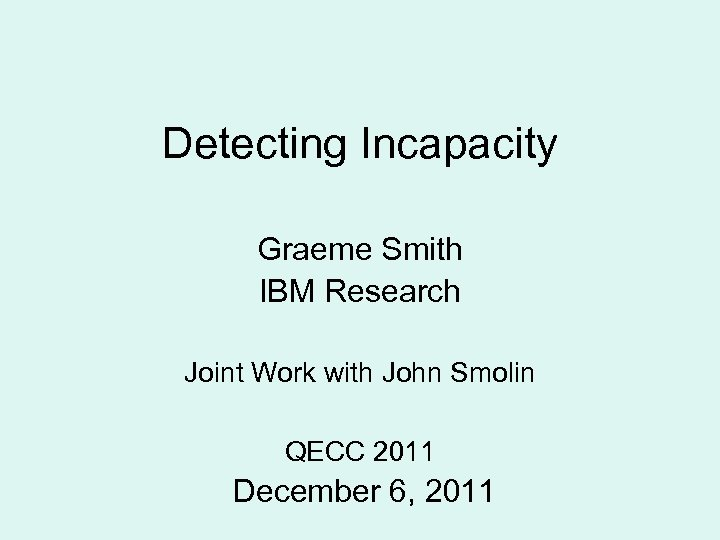Detecting Incapacity Graeme Smith IBM Research Joint Work with John Smolin QECC 2011 December 6, 2011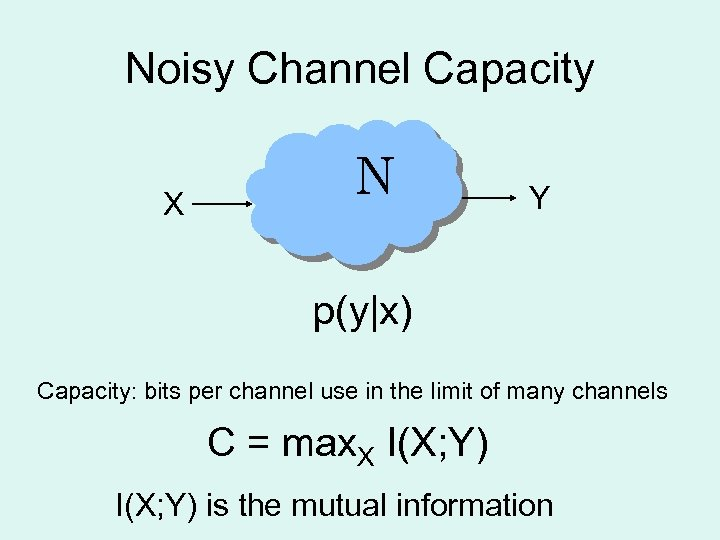Noisy Channel Capacity X N Y p(y|x) Capacity: bits per channel use in the limit of many channels C = max. X I(X; Y) is the mutual information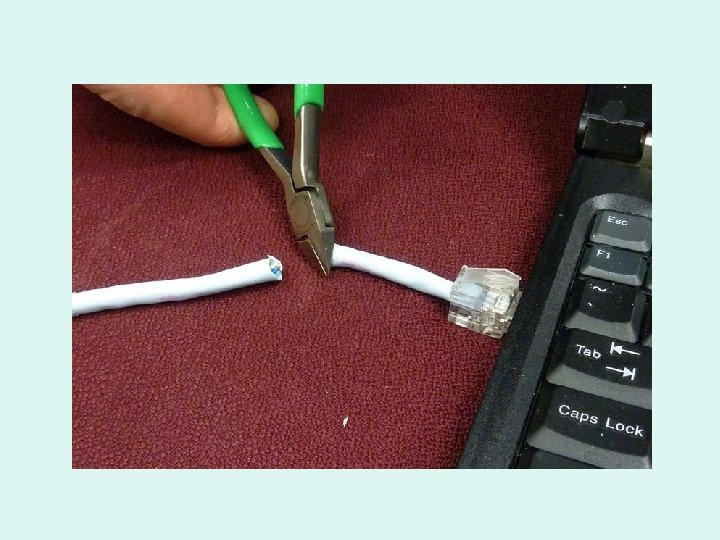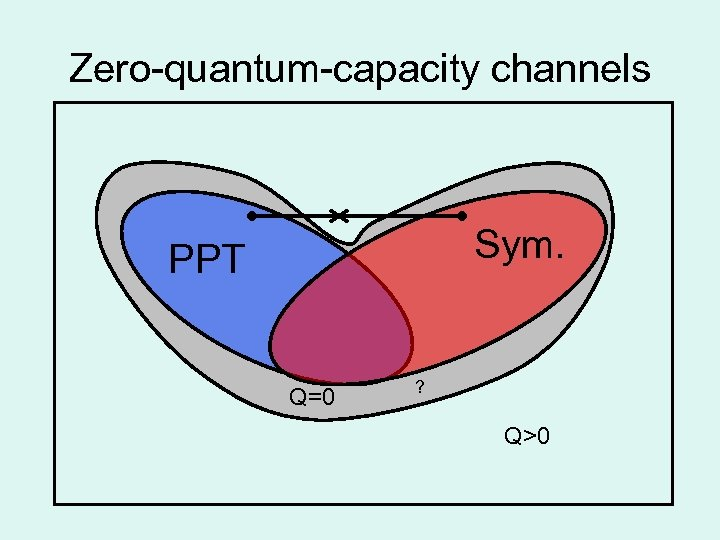Zero-quantum-capacity channels Sym. PPT Q=0 ? Q>0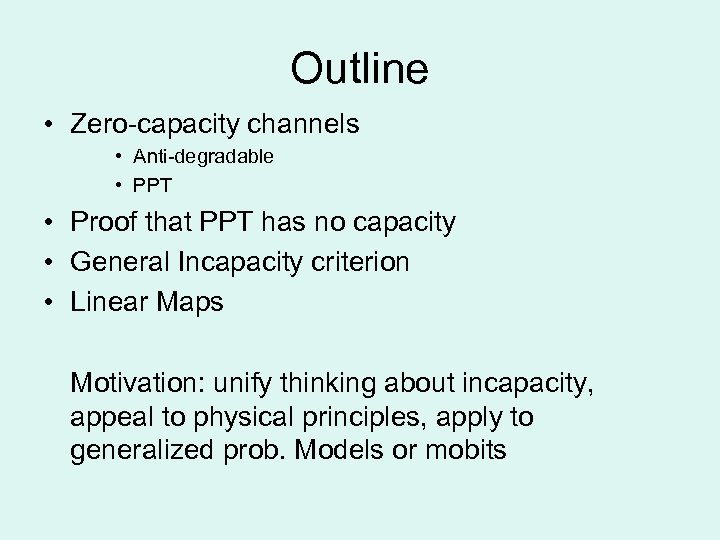Outline • Zero-capacity channels • Anti-degradable • PPT • Proof that PPT has no capacity • General Incapacity criterion • Linear Maps Motivation: unify thinking about incapacity, appeal to physical principles, apply to generalized prob. Models or mobits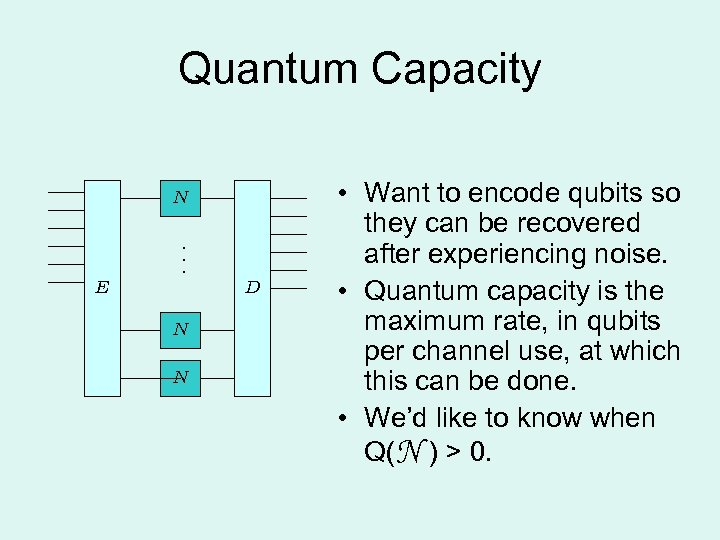Quantum Capacity N. . . E D N N • Want to encode qubits so they can be recovered after experiencing noise. • Quantum capacity is the maximum rate, in qubits per channel use, at which this can be done. • We’d like to know when Q(N ) > 0.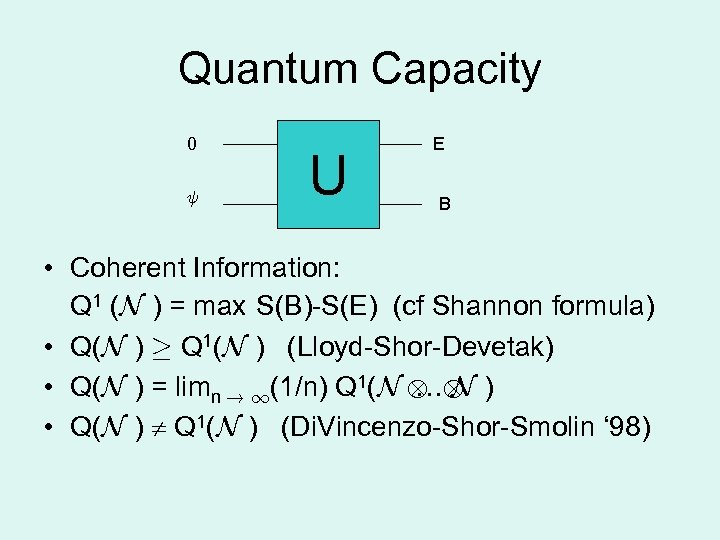Quantum Capacity 0 Ã U E B • Coherent Information: Q 1 (N ) = max S(B)-S(E) (cf Shannon formula) • Q(N ) ¸ Q 1(N ) (Lloyd-Shor-Devetak) • Q(N ) = limn ! 1(1/n) Q 1(N N ) … • Q(N ) Q 1(N ) (Di. Vincenzo-Shor-Smolin ‘ 98)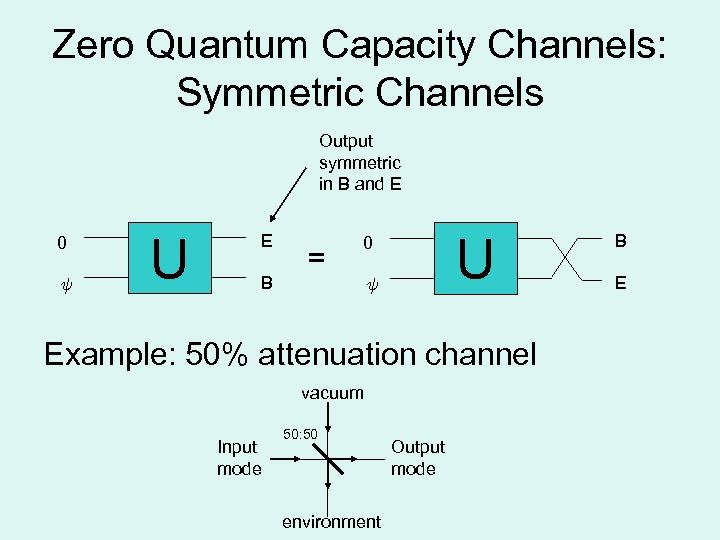Zero Quantum Capacity Channels: Symmetric Channels Output symmetric in B and E 0 Ã U E B = U 0 Ã Example: 50% attenuation channel vacuum Input mode 50: 50 environment Output mode B E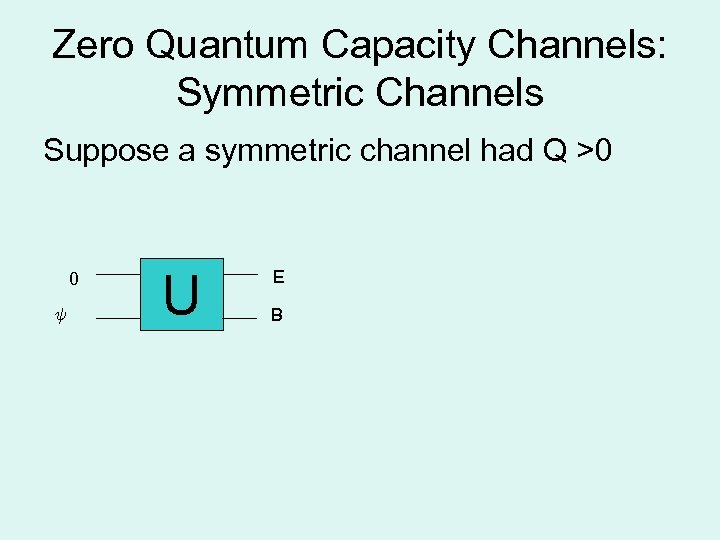Zero Quantum Capacity Channels: Symmetric Channels Suppose a symmetric channel had Q >0 0 Ã U E B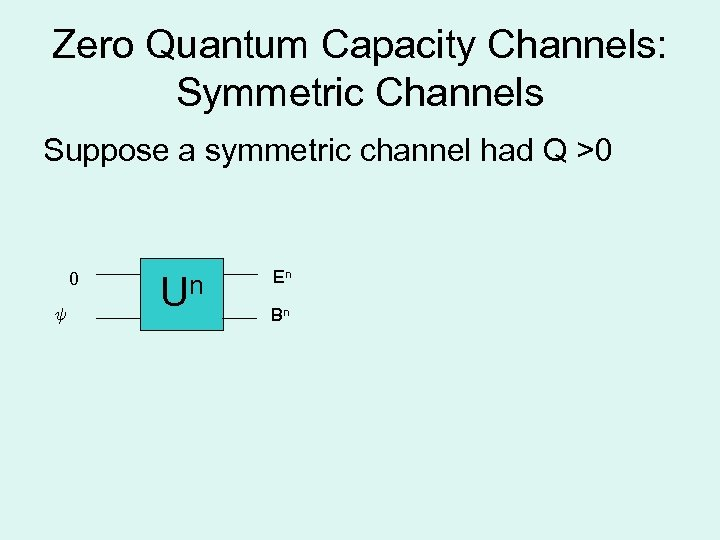Zero Quantum Capacity Channels: Symmetric Channels Suppose a symmetric channel had Q >0 0 Ã Un En Bn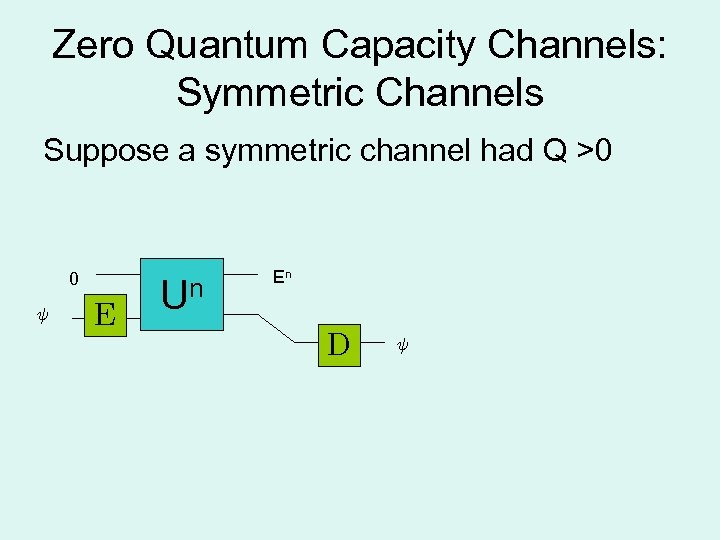Zero Quantum Capacity Channels: Symmetric Channels Suppose a symmetric channel had Q >0 0 Ã E Un En D Ã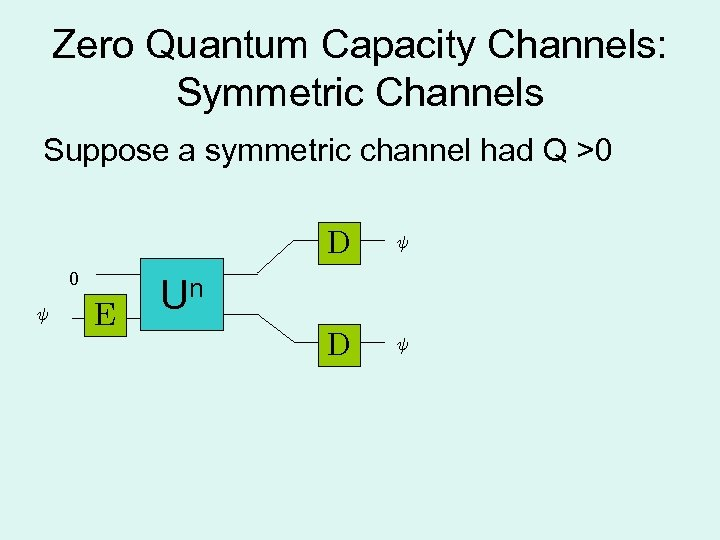Zero Quantum Capacity Channels: Symmetric Channels Suppose a symmetric channel had Q >0 D 0 Ã E Ã D Ã Un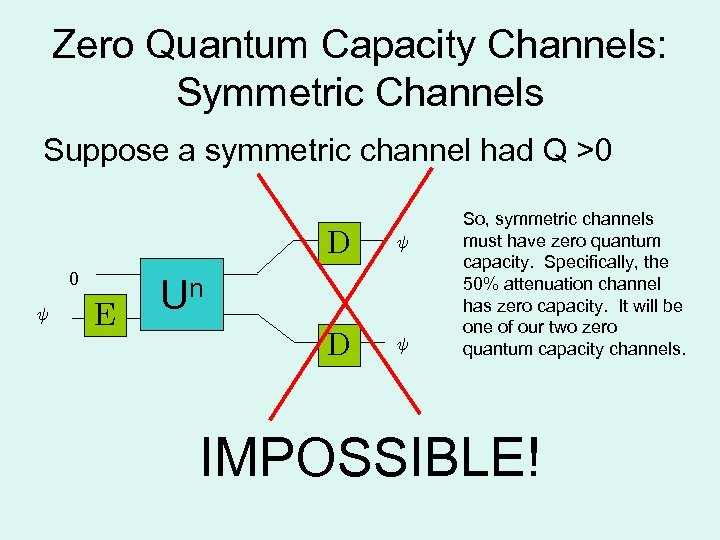Zero Quantum Capacity Channels: Symmetric Channels Suppose a symmetric channel had Q >0 D 0 Ã E Ã D Ã Un So, symmetric channels must have zero quantum capacity. Specifically, the 50% attenuation channel has zero capacity. It will be one of our two zero quantum capacity channels. IMPOSSIBLE!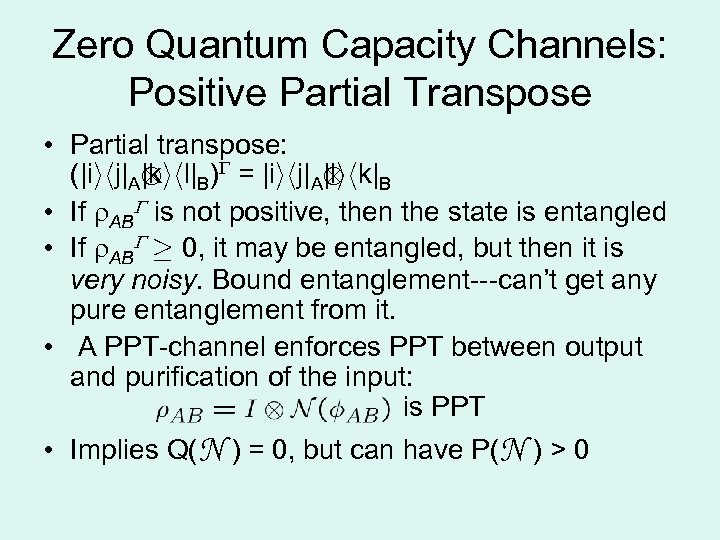Zero Quantum Capacity Channels: Positive Partial Transpose • Partial transpose: (|iihj|A B) = |iihj|A B |kihl| |lihk| • If AB is not positive, then the state is entangled • If AB ¸ 0, it may be entangled, but then it is very noisy. Bound entanglement---can’t get any pure entanglement from it. • A PPT-channel enforces PPT between output and purification of the input: is PPT • Implies Q(N ) = 0, but can have P(N ) > 0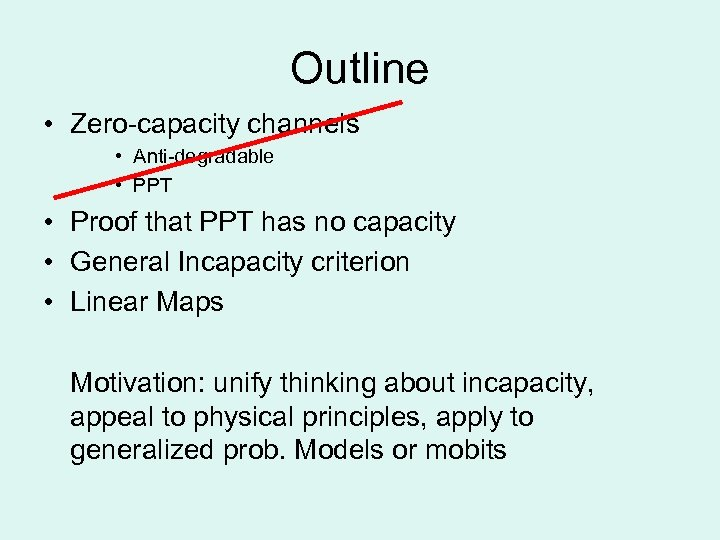Outline • Zero-capacity channels • Anti-degradable • PPT • Proof that PPT has no capacity • General Incapacity criterion • Linear Maps Motivation: unify thinking about incapacity, appeal to physical principles, apply to generalized prob. Models or mobits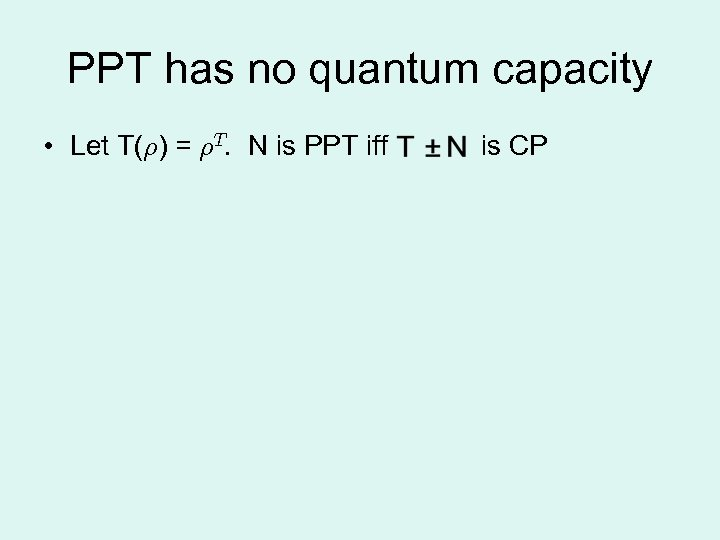PPT has no quantum capacity • Let T(½) = ½T. N is PPT iff is CP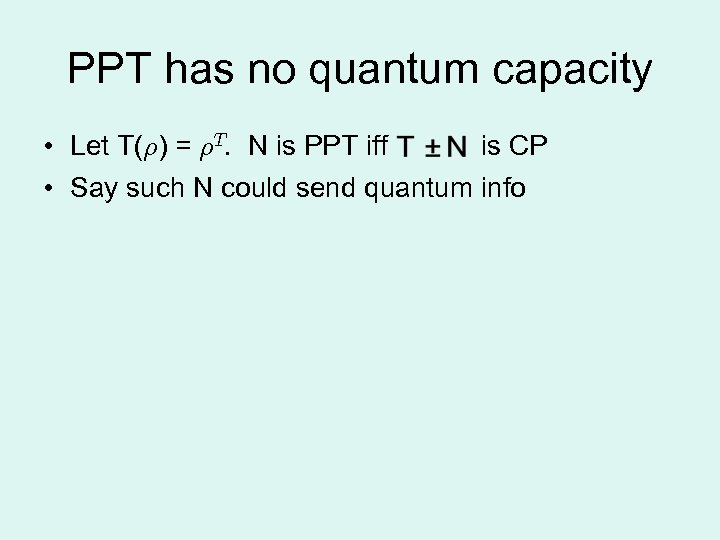PPT has no quantum capacity • Let T(½) = ½T. N is PPT iff is CP • Say such N could send quantum info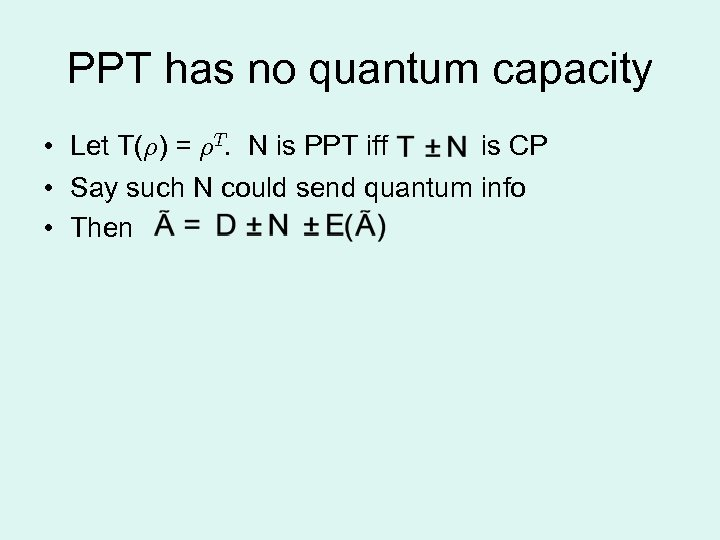PPT has no quantum capacity • Let T(½) = ½T. N is PPT iff is CP • Say such N could send quantum info • Then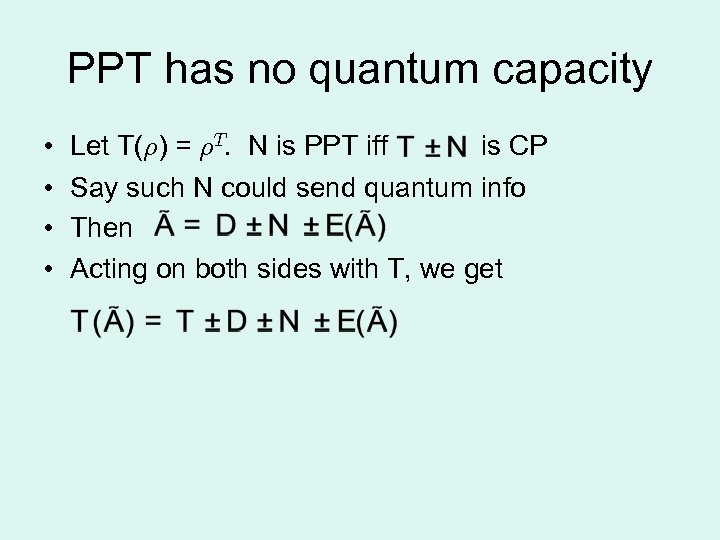PPT has no quantum capacity • • Let T(½) = ½T. N is PPT iff is CP Say such N could send quantum info Then Acting on both sides with T, we get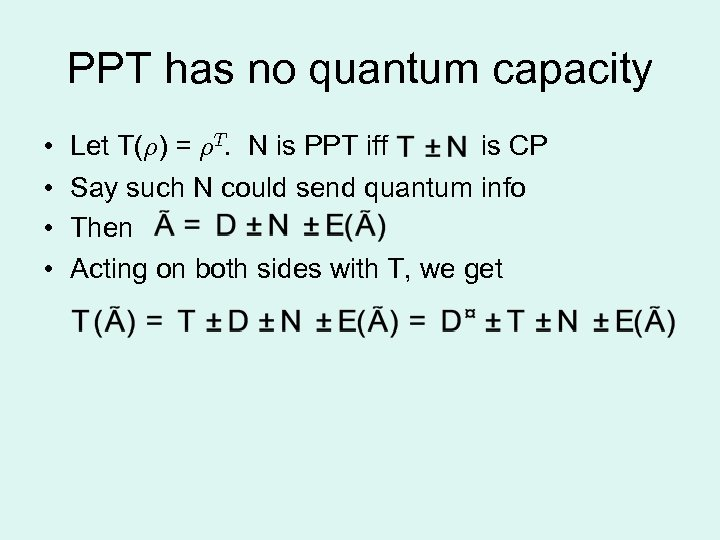PPT has no quantum capacity • • Let T(½) = ½T. N is PPT iff is CP Say such N could send quantum info Then Acting on both sides with T, we get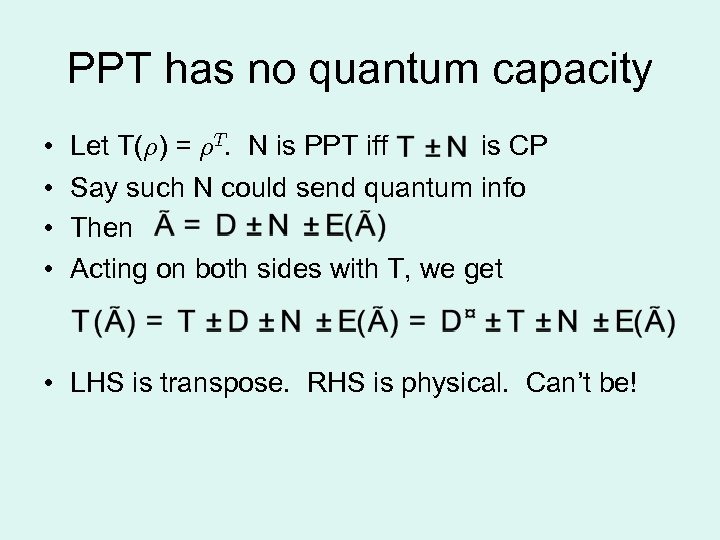PPT has no quantum capacity • • Let T(½) = ½T. N is PPT iff is CP Say such N could send quantum info Then Acting on both sides with T, we get • LHS is transpose. RHS is physical. Can’t be!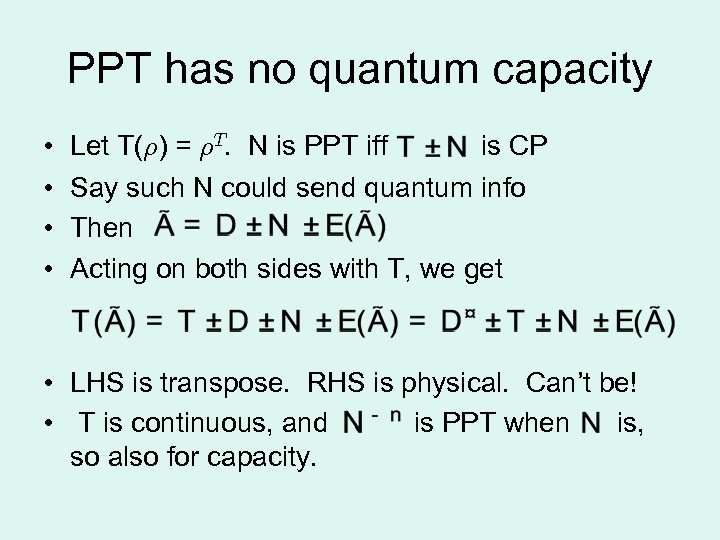PPT has no quantum capacity • • Let T(½) = ½T. N is PPT iff is CP Say such N could send quantum info Then Acting on both sides with T, we get • LHS is transpose. RHS is physical. Can’t be! • T is continuous, and is PPT when is, so also for capacity.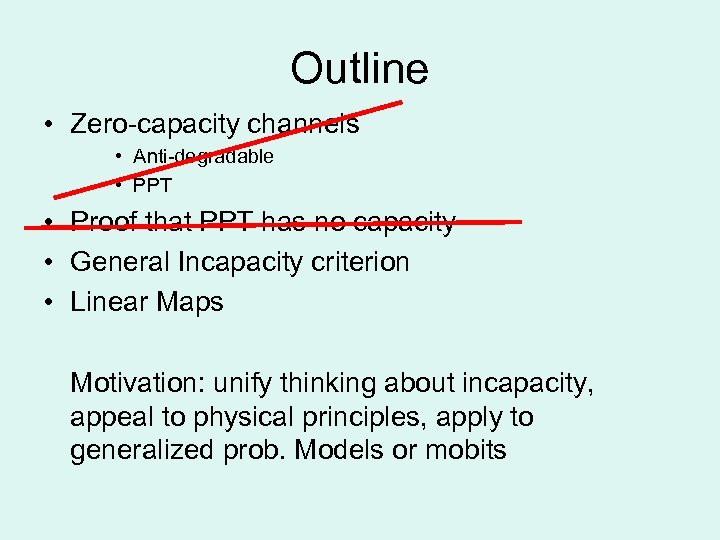Outline • Zero-capacity channels • Anti-degradable • PPT • Proof that PPT has no capacity • General Incapacity criterion • Linear Maps Motivation: unify thinking about incapacity, appeal to physical principles, apply to generalized prob. Models or mobits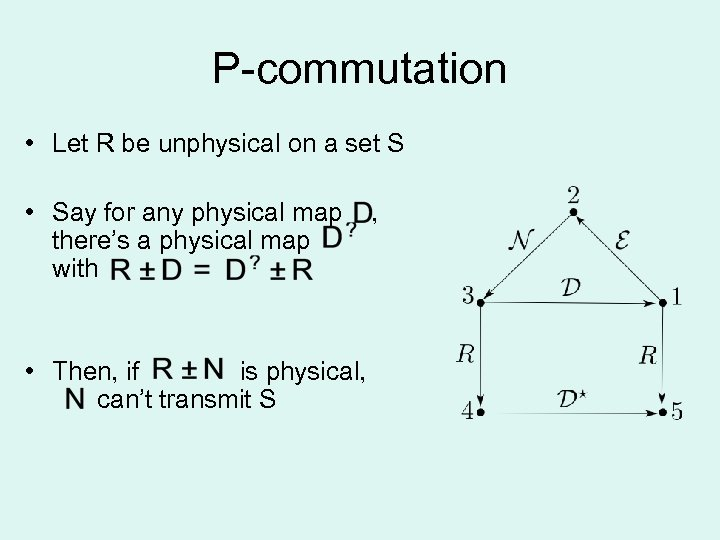P-commutation • Let R be unphysical on a set S • Say for any physical map there’s a physical map with • Then, if is physical, can’t transmit S ,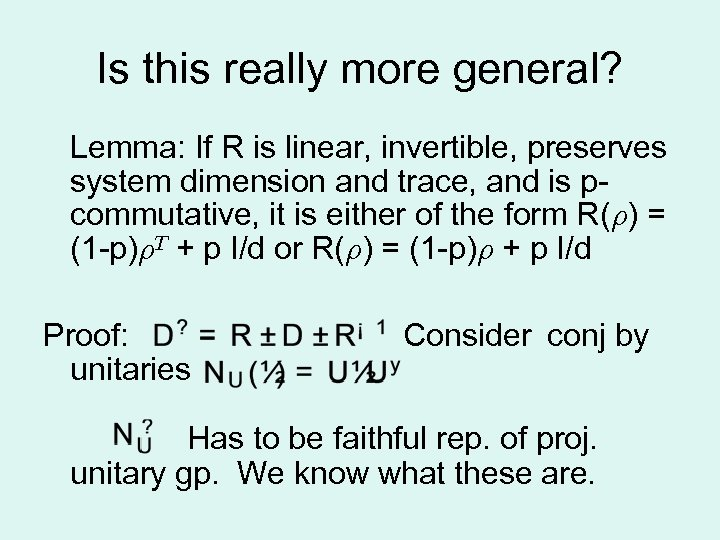Is this really more general? Lemma: If R is linear, invertible, preserves system dimension and trace, and is pcommutative, it is either of the form R(½) = (1 -p)½T + p I/d or R(½) = (1 -p)½ + p I/d Proof: unitaries Consider conj by Has to be faithful rep. of proj. unitary gp. We know what these are.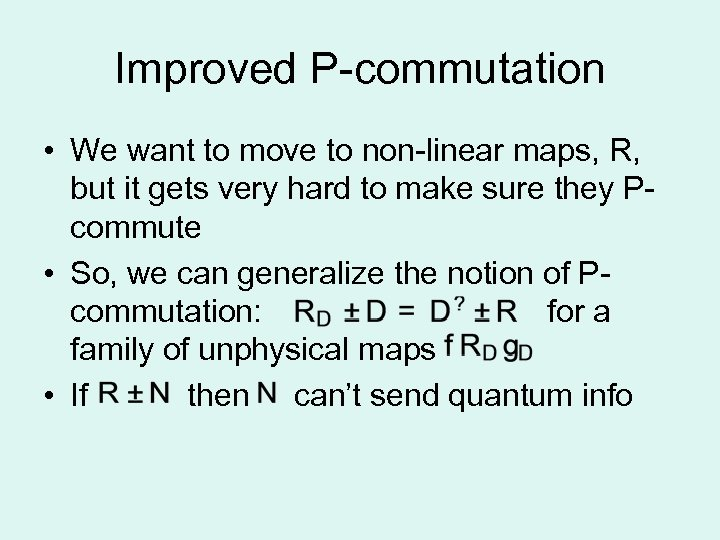Improved P-commutation • We want to move to non-linear maps, R, but it gets very hard to make sure they Pcommute • So, we can generalize the notion of Pcommutation: for a family of unphysical maps • If then can’t send quantum info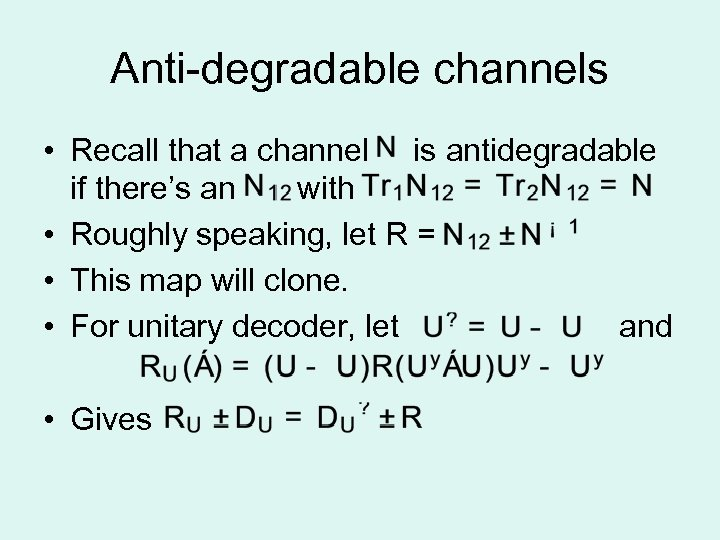Anti-degradable channels • Recall that a channel is antidegradable if there’s an with • Roughly speaking, let R = • This map will clone. • For unitary decoder, let and • Gives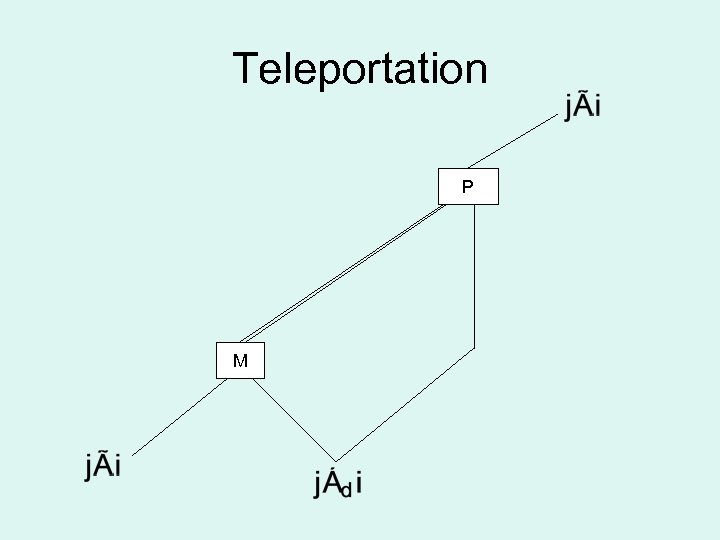Teleportation P M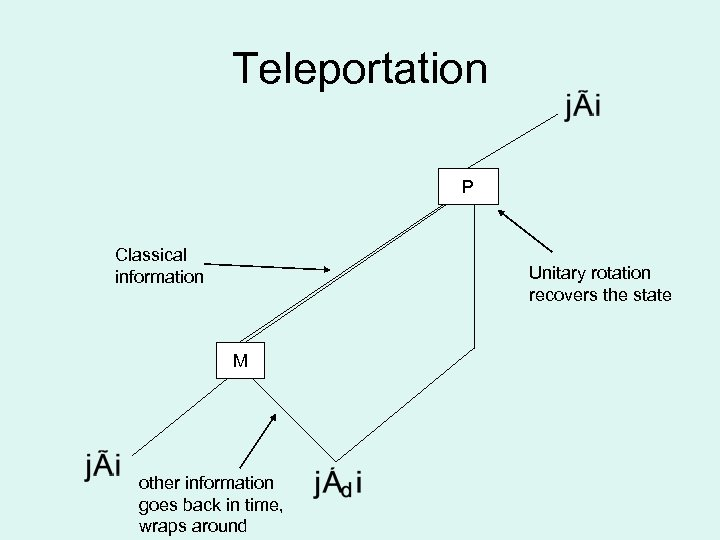Teleportation P Classical information Unitary rotation recovers the state M other information goes back in time, wraps around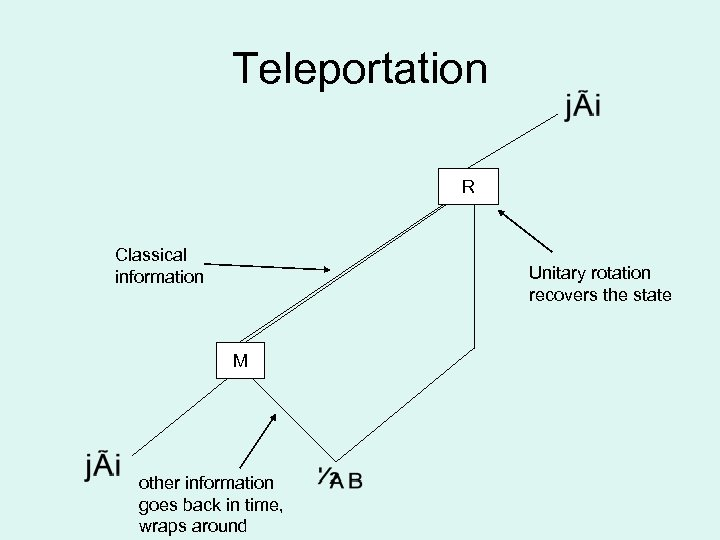Teleportation R Classical information Unitary rotation recovers the state M other information goes back in time, wraps around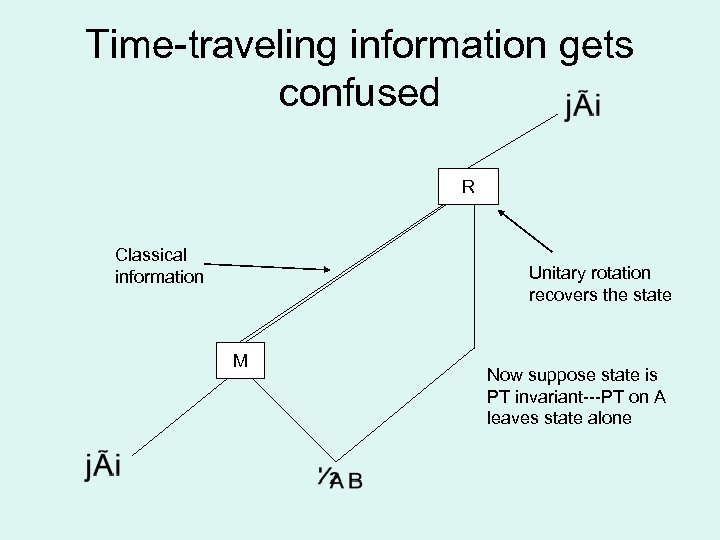Time-traveling information gets confused R Classical information Unitary rotation recovers the state M Now suppose state is PT invariant---PT on A leaves state alone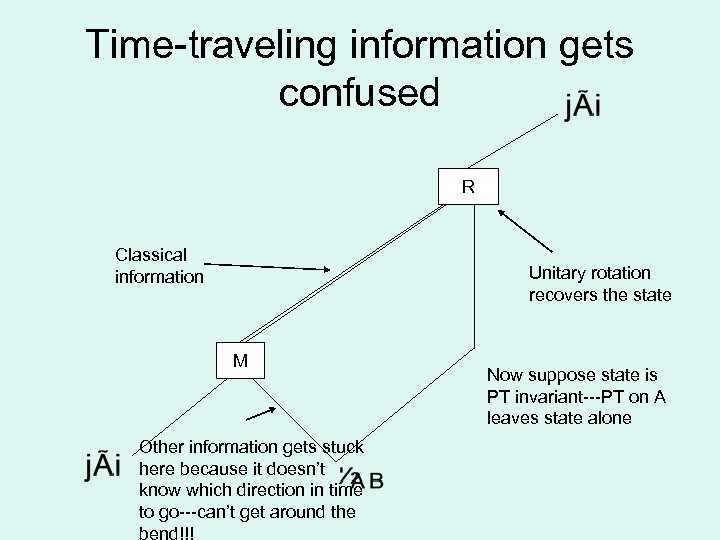Time-traveling information gets confused R Classical information Unitary rotation recovers the state M Other information gets stuck here because it doesn’t know which direction in time to go---can’t get around the bend!!! Now suppose state is PT invariant---PT on A leaves state alone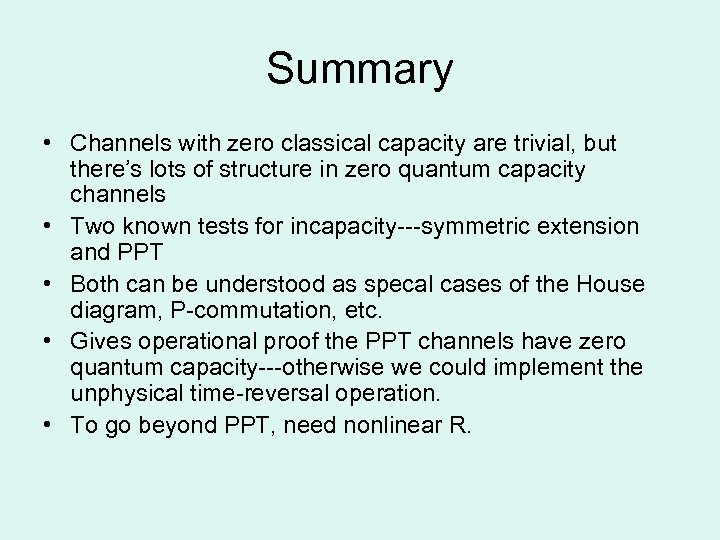Summary • Channels with zero classical capacity are trivial, but there’s lots of structure in zero quantum capacity channels • Two known tests for incapacity---symmetric extension and PPT • Both can be understood as specal cases of the House diagram, P-commutation, etc. • Gives operational proof the PPT channels have zero quantum capacity---otherwise we could implement the unphysical time-reversal operation. • To go beyond PPT, need nonlinear R.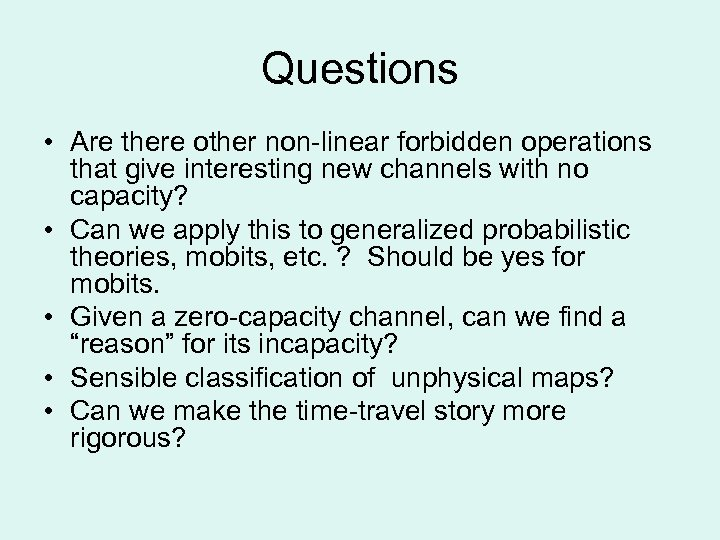Questions • Are there other non-linear forbidden operations that give interesting new channels with no capacity? • Can we apply this to generalized probabilistic theories, mobits, etc. ? Should be yes for mobits. • Given a zero-capacity channel, can we find a “reason” for its incapacity? • Sensible classification of unphysical maps? • Can we make the time-travel story more rigorous?